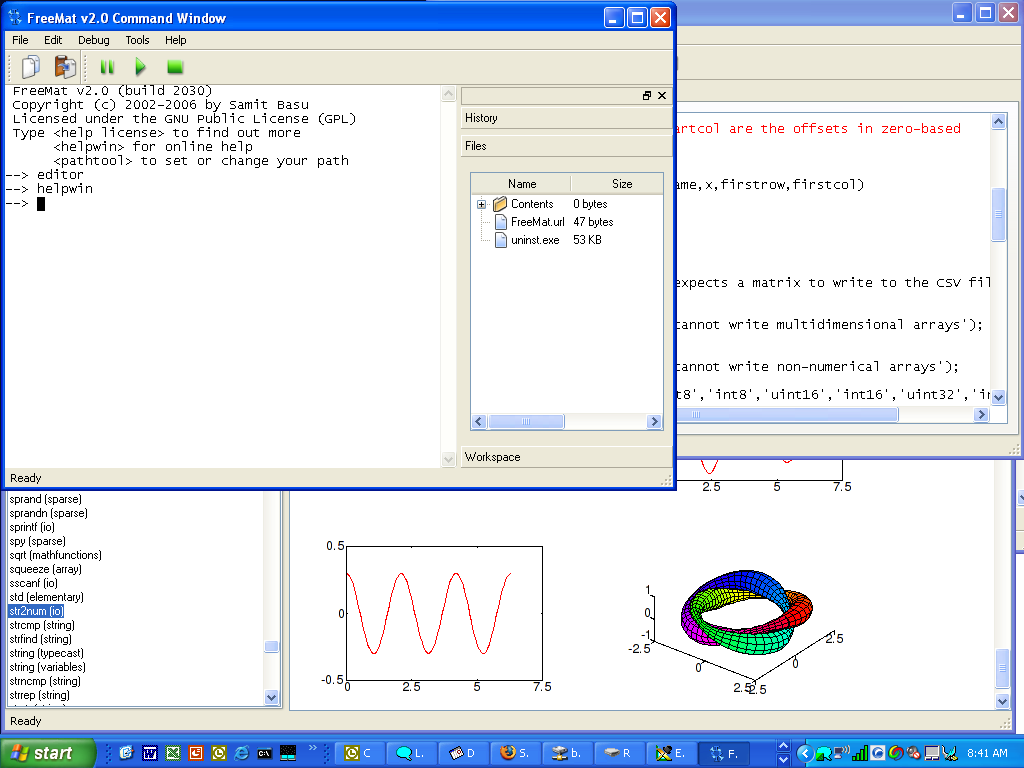## FreeMat for Windows 4.2 Full ScreenshotFreeMat is a free environment for rapid engineering and scientific prototyping and data processing. It is similar to commercial systems such as MATLAB from Mathworks, and IDL from Research Systems, but is Open Source. FreeMat is available under the GPL license.

In addition to supporting many MATLAB functions and some IDL functionality, FreeMat features a codeless interface to external C, C++, and Fortran code. ... read more

#### Octave 5.1.0John W. Eaton

High-level interpreted language, primarily intended for numerical computations. Free download of Octave 5.1.0#### Yorick for Mac OS X 2.2.0.4Yorick.sf.net

Yorick is an interpreted programming language for scientific simulations. Free download of Yorick for Mac OS X 2.2.0.4#### Yorick for Windows 2.2.0.4Yorick.sf.net

Yorick is an interpreted programming language for scientific simulations. Free download of Yorick for Windows 2.2.0.4#### Math Studio 3.0GraphNow

Dynamic geometry tool for school math teaching and studying.. Free download of Math Studio 3.0

#### The R Commander 2.5-3John Fox

A Basic-Statistics GUI for R. Free download of The R Commander 2.5-3

#### Regression Analysis - CurveFitter 4.5.22Institute of Mathematics and Statistics

CurveFitter performs regression analysis to estimate values of parameters.. Free download of Regression Analysis - CurveFitter 4.5.22

#### Scientific Calculator - ScienCalc 1.3.22Institute of Mathematics and Statistics

ScienCalc is a convenient and powerful scientific calculator.. Free download of Scientific Calculator - ScienCalc 1.3.22

#### Equation graph plotter - EqPlot 1.3.37Institute of Mathematics and Statistics

EqPlot plots 2D graphs from complex equations.. Free download of Equation graph plotter - EqPlot 1.3.37

#### Desktop Calculator - DesktopCalc 2.1.22Institute of Mathematics and Statistics

DesktopCalc is an enhanced, easy-to-use and powerful scientific calculator. Free download of Desktop Calculator - DesktopCalc 2.1.22

#### Multipurpose Calculator - MultiplexCalc 5.4.22Institute of Mathematics and Statistics

MultiplexCalc is a multipurpose and comprehensive desktop calculator for Windows. Free download of Multipurpose Calculator - MultiplexCalc 5.4.22